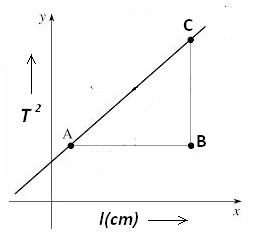Simple pendulum

# Objective

Our objectives are;

• To plot a L-T2 graph using a simple pendulum.
• To find the effective length of the simple pendulum for a given time period using the graph.
• To calculate the acceleration due to gravity at a place.

# The Theory

### What is a Simple Pendulum?

An ideal simple pendulum consists of a heavy point mass (called bob) tied to one end of a perfectly inextensible, flexible and weightless string. In practice, we make it by tying a metallic spherical bob to a fine cotton stitching thread.

### Length of a Simple Pendulum

The distance between the point of suspension of the pendulum and its Centre of Gravity (C.G.), which is the C.G. of the bob, is called the length of the simple pendulum. It is represented using the aphabet ( l ).

### Time Period of a Simple Pendulum

Time period is the time taken by the bob of the simple pendulum to make one complete oscillation. It is represented by the letter T.

### Finding the acceleration due to gravity

The time period of a simple pendulum depends on the length of the pendulum (l) and the acceleration due to gravity (g), which is expressed by the relation,For small amplitude of oscillations,ie;If we know the value of l and T, we can calculate the acceleration due to gravity, g at that place.

### The L-T2 graph

We can plot a graph between l and T2 by taking l along the X axis and T2 along the Y axis. The graph is a straight line.From the graph,## Learning Outcomes

• Students get to know about the simple pendulum.
• Students understand the relation between the length of the pendulum, time period and the acceleration due to gravity at a place.
• They get an idea about the importance of the l-T2 graph.